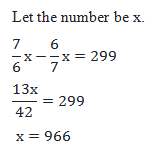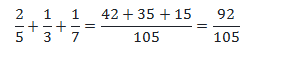# SSC CGL 2018 Practice Test Papers | Quantitative Aptitude (Day-48)

Dear Aspirants, Here we have given the Important SSC CGL Exam 2018 Practice Test Papers. Candidates those who are preparing for SSC CGL 2018 can practice these questions to get more confidence to Crack SSC CGL 2018 Examination.

[WpProQuiz 3512]

Click “Start Quiz” to attend these Questions and view Explanation

1) The average age of class A is 20 % more than that of class B and number of students in class A is 80% of that of class B. If the average of all students is 9.8 then what is the average age of class B?

a) 10

b) 11

c) 9

d) 6

2) A and B together can do a work in 30 days. They worked for 20 days and then B left the work. The remaining work was done by A in 20 days then how long B alone can do half of the work?

a) 15

b) 20

c) 30

d) 40

3) Harish bought a book for Rs.485 and sold it at 20% loss. By using that amount he bought another book and sold it at 15% profit. Then overall profit/loss amount is:

a) 38.8% loss

b) 40.2% profit

c) 39.7 % loss

d) 46.8% profit

4) Train A of length 120 m can cross a platform of length 240 m in 18 second the ratio of speed of train A and Train B is 4 : 5. Then find the length of Train B if train B can cross a pole in 12 seconds.

a) 100 m

b) 300 m

c) 20 m

d) 250 m

5) The average monthly salary of 550 workers in a factory is Rs. 360. The average monthly salary of officers is Rs. 500 and average monthly salary of the other workers is Rs. 225. Find the no. of other workers

a) 280

b) 270

c) 250

d) 220

6) If the sum of circumference of a circle and the circumference of a semicircle which radius is same, is 400cm. Then find the radius of another circle whose radius is 100% more than R?

a) 70

b) 35

c) 50

d) 55

7) There are four consecutive even integers. Sum of the reciprocals of the first two numbers is (7/24). Find the reciprocal of second largest number of the series.

a) 1/12

b) 1/14

c) 1/10

d) 1/8

8) Five people visit A, B, C, D and E visit a temple at intervals of 1, 2, 3 4 and 5 days respectively. If they all together visit the temple today, then after how many days will they visit the temple on the same day for the next time?

a) 30

b) 36

c) 120

d) 60

9) A was asked to find 6/7 of a number but instead he multiplied it by 7/6 As a result he got an answer which was 299 more than the correct answer. What was the number?

a) 922

b) 966

c) 754

d) 841

10) 2/5 + 1/3 + 1/7 = ?

a) 112/ 95

b) 92/105

c) 129/105

d) 29/105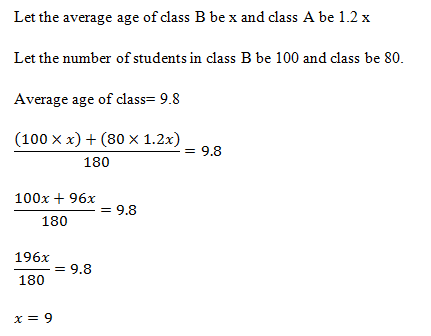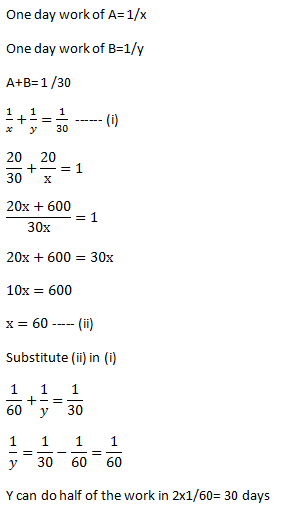CP of 1st book = 485

SP of 1st book = 80/100 × 485 = 388

Loss = 485 – 388 = 97

Cp of 2nd book = 388

Sp of 2nd book = 388×115/100 = 446.2

Profit = 446.2 – 388 = 58.2

Overall loss= 97 – 58.2 = 38.8

(Or) CP of 1st book = 485

SP of 1st book= 80/100×485 = 388

Cp of 2nd book =388

Sp of 2nd book = 388 × 115/100 = 446.2

Loss= 485 – 446.2 = 38.8

Speed of train A, S1= Distance /time

= (120+240)/18=20 m/s

Speed of train A: Speed of Train B= 4: 5

Speed of train B= 20/4×5=25m/s

25 = Length of train B/12

Length of train B= 300 m

Total Salary of workers = 550×360=198000

Number of officers = x

Average salary of officers = 500

Total salary of officers = 500x

Number of other workers = (550 – x)

Average salary of other workers = 225

Total salary of other workers = (550 – x) 225

(550 – x) 225+500x = 198000

X= 270

Number of other workers = 550 – 270 = 280

Circumference of circle = 2πr

Circumference of semicircle= πr+2r

2πr + πr+2r =400

3×22/7xr+2r=400

66r/7+2r=400

80r/7=400

80r=2800

R = 35

100 %

200% of R = 200/100×35 = 70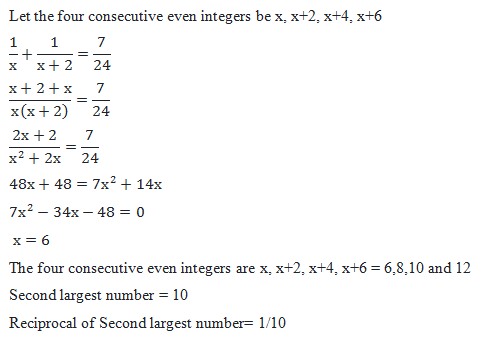LCM of 1,2,3,4 and 5=60

The five people will visit the temple after 60 days